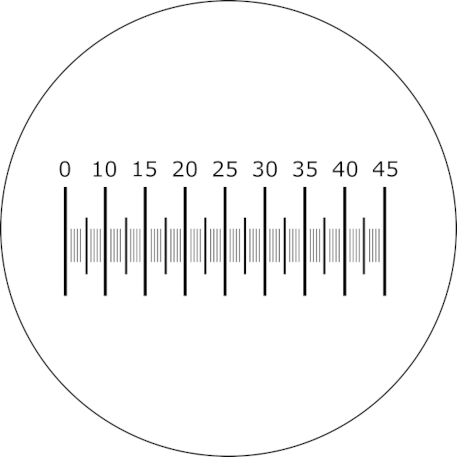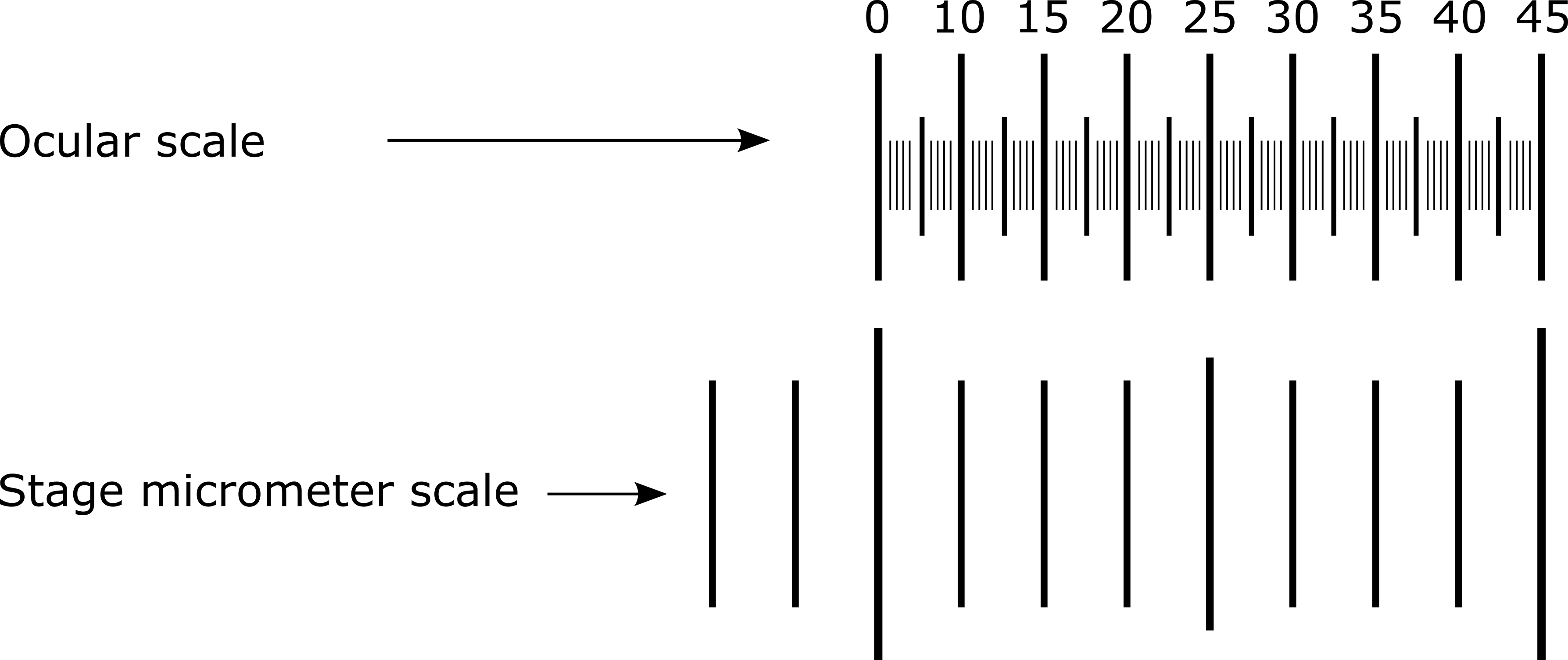## 17.3 Method 2: Ocular Micrometer

The ocular micrometer is inserted into one of the eyepieces of the microscope and looks like a ruler.Caption The Ocular Micrometer.
Image produced by Mathew Vis-Dunbar licensed under CC BY-NC-SA 4.0

The distance between the markings on the micrometer are used to measure objects in the field of view and represent different sizes of increments at different magnifications because the diameter of the field of view decreases as the magnification increases.

In order to determine the size represented by the increments at different magnifications, the ocular micrometer must be calibrated against a stage micrometer (a special slide containing a scale marked off in 10$$\mu$$m increments).

### Calibrating the Ocular Micrometer

To calibrate the ocular micrometer, follow these steps:

1. Look through the microscope eyepieces and note the ocular micrometer.
2. Obtain a stage micrometer and bring the scale into focus using the 40x objective (remember to focus it first using the 4x and 10x objectives).
3. Rotate the ocular lens tube until the markings of the ocular micrometer are lined up parallel to those of the stage micrometer and superimposed on them.
4. Use the mechanical stage adjustment knob to move the stage micrometer so that the lines of both micrometers coincide at the left edge of the field.Caption Calibration of the ocular micrometer. Note that this diagram is only an example and will not necessarily reflect the alignment you will see under your own microscope.
Image produced by Mathew Vis-Dunbar licensed under [CC BY-NC-SA 4.0]

1. Starting with the lowest magnification objective, carefully observe and count the number of increments on the ocular micrometer that correspond to a single 10$$\mu$$m division (the smallest division on the stage micrometer) of the stage micrometer. Repeat this for each objective lens.
2. Calculate the size of one ocular micrometer unit using the following formula:

$o=\frac{10\mu m}{u}$

where

• $$o$$ = size of 1 ocular micrometer unit (in $$\mu$$m)
• 10$$\mu m$$ is the width of 1 stage micrometer unit
• $$u$$ = # of ocular micrometer units in 1 stage micrometer unit

Example: Using a particular objective lens, you find that there are 5 ocular units in one stage micrometer unit. By using the formula above, you can then determine that each ocular micrometer unit for that objective is equal to 2 $$\mu$$m. See the calculations below:

$o=\frac{10\mu m}{5 ocular micrometer units}=2\mu m$

1. Now you can calculate the size of a specimen by counting the number of ocular micrometer increments spanned by the specimen and multiplying it by the width of the ocular micrometer unit at that specific magnification. If your specimen is longer than it is wide, do this for both the width and the length of your specimen.

Make sure to measure more than one specimen and report the range of sizes for each dimension to account for the natural variation that occurs in the population. For example, a bacillus-shaped bacterium that ranges between 1 to 5 $$\mu m$$ in width and 4 to 10 $$\mu m$$ in length would be expressed as 1-5$$\mu m$$ x 4-10 $$\mu m$$.